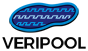### ProfileHome News Activity About/Contact Major Tools Dinotrace Verilator Verilog-mode Verilog-Perl Other Tools IPC::Locker Parallel::Forker Voneline General Info Papers

## Issue #1321

### indentation of coverpoint is incorrect if coverpoint expression is a concatenation

Status:
Confirmed
Priority:
Normal
Assignee:
-
Category:
Indents
% Done:

0%

Description

Coverpoints on curly-brace concatenations indent incorrectly, and accumulate indent level.

```module m;
bit a, b, c;
covergroup g;
cp_ab: coverpoint {a,b} {
bins one = {1};
bins two = {2};
}
cp_bc: coverpoint {b,c} {
bins one = {1};
bins two = {2};
}
endgroup
endmodule
```
Desired/correct indenting is seen if an iff clause is added to the coverpoints:
```module m;
bit a, b, c;
covergroup g;
cp_ab: coverpoint {a,b} iff (1) {
bins one = {1};
bins two = {2};
}
cp_bc: coverpoint {b,c} iff (1) {
bins one = {1};
bins two = {2};
}
endgroup
endmodule
```
Verilog-mode version is 2018-05-26-be4eda3-vpo.

### History

#### #1 Updated by Wilson Snyderover 1 year ago

• Category set to Indents
• Status changed from New to Confirmed

Yes, looks wrong. The indentation code is presently without a major maintainer, so it might be a while before this is fixed unless you can provide a patch.

#### #2 Updated by Robert Swanover 1 year ago

The following is working for me:

verilog-mode version 2018-05-26-be4eda3-vpo:

```module m;
bit[0:0] a, b, c;
covergroup g;
cp_ab: coverpoint {a,b} {
bins one = {1};
bins two = {2};
}

cp_ab_if_c: coverpoint {a,b} iff c {
bins one = {1};
bins two = {2};
}

cp_ab_if_c_slice: coverpoint {a,b} iff c {
bins one = {1};
bins two = {2};
}

cp_a_if_bc: coverpoint {a,b} iff {b,c} {
bins one = {1};
bins two = {2};
}

cp_a_slice : coverpoint a {
bins one = {1};
bins two = {2};
}

cp_a_slice_if_b : coverpoint a iff b {
bins one = {1};
bins two = {2};
}

cp_a_if_b_slice : coverpoint a iff b {
bins one = {1};
bins two = {2};
}

cp_a_slice_if_b_slice : coverpoint a iff b {
bins one = {1};
bins two = {2};
}
endgroup
endmodule
```

Patch:

```diff --git a/verilog-mode.el-2018-05-26-be4eda3-vpo b/verilog-mode.el-indent-fix
index f7f757f..a5a3617 100644
--- a/verilog-mode.el-2018-05-26-be4eda3-vpo
+++ b/verilog-mode.el-indent-fix
@@ -6450,6 +6450,34 @@ Return >0 for nested struct."
"\\(?:\\w+\\s-*:\\s-*\\)?\\(coverpoint\\|cross\\)"
"\\|with\\)\\>\\|" verilog-in-constraint-re)))
(setq pass 1)))))
+
+          ;; step backwards over coverpoint/iff on concatenation/slice
+          (catch 'coverpoint-or-iff
+            (dotimes (test-coverpoint-or-iff 4)
+              (if (looking-at "coverpoint")
+                  (progn
+                    (verilog-beg-of-statement)
+                    (setq pass 1)
+                    (throw 'coverpoint-or-iff nil)))
+              (if (equal (char-after) ?\})
+                  (progn
+                    (forward-char 1)
+                    (backward-list)
+                    (verilog-backward-sexp))
+                (if (equal (char-after) ?\[)
+                    (progn
+                      (verilog-backward-token)
+                      (verilog-backward-sexp)
+                      )
+                  (if (or (looking-at "iff"))
+                      (progn
+                        (verilog-backward-ws&directives)
+                        (verilog-backward-token))
+                    (if (eq pass 0)
+                        (progn
+                          (verilog-backward-ws&directives)
+                          (verilog-backward-sexp))))))))
+
(if (eq pass 0)
(progn (goto-char pt) nil) 1)))
;; not

```
Patched:
```module m;
bit[0:0] a, b, c;
covergroup g;
cp_ab: coverpoint {a,b} {
bins one = {1};
bins two = {2};
}

cp_ab_if_c: coverpoint {a,b} iff c {
bins one = {1};
bins two = {2};
}

cp_ab_if_c_slice: coverpoint {a,b} iff c {
bins one = {1};
bins two = {2};
}

cp_a_if_bc: coverpoint {a,b} iff {b,c} {
bins one = {1};
bins two = {2};
}

cp_a_slice : coverpoint a {
bins one = {1};
bins two = {2};
}

cp_a_slice_if_b : coverpoint a iff b {
bins one = {1};
bins two = {2};
}

cp_a_if_b_slice : coverpoint a iff b {
bins one = {1};
bins two = {2};
}

cp_a_slice_if_b_slice : coverpoint a iff b {
bins one = {1};
bins two = {2};
}
endgroup
endmodule

```

#### #3 Updated by Wilson Snyderover 1 year ago

• Status changed from Confirmed to Assigned
• Assignee set to Robert Swan

Great work this passes on your new test, but if you could please download the git tree and try the self tests this fails with an error "wrong type argument: consp"

Also a nit, in Lisp rather than this

``````(if (a)
(foo)
(if (b)
(bar)
(if (c)
(baz))))``````

do this

``````(cond
((a)
(foo))
((b)
(bar))
((c)
(baz)))``````

If not clear I'll clean it up for you.

#### #4 Updated by Wilson Snyderabout 1 month ago

• Status changed from Assigned to Confirmed
• Assignee deleted (Robert Swan)

Still awaiting a patch+test.

Also available in: Atom